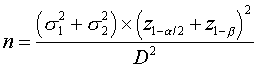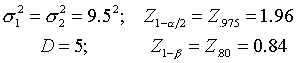# Sample size calculation example

## 2004-05-20

I received a question in Hong Kong about how to double check a power calculation in a paper by Tugwell et all in the 1995 NEJM [Medline]](http://www.ncbi.nlm.nih.gov/entrez/query.fcgi?cmd=Retrieve&db=pubmed&dopt=Abstract&list_uids=7791814). In the paper, they state that

With the tender-joint count used as the primary outcome, a sample of 75 patients per group was needed in order to have a 5 percent probability of a Type I error and a power of 80 percent to detect a difference of 5 tender joints between groups, with a standard deviation of 9.5, and to allow for a 25 percent dropout rate.

and the formula for estimating the sample size would bewhere D represents the minimum clinically relevant difference. This formula assumes that you have two parallel groups and you are comparing them with a continuous outcome variable using a two-sided t-test. There are several variations on this formula, but they all give the same answer. Extracting the information from the text shown above, you would getUsing these numbers, I get an estimated sample size of 56.60. In order to allow for dropouts, divide this number by 0.75 to get 75.47. I would round this number up, but could not criticize someone who rounded it downward.

You can find an earlier version of this page on my original website.